# Selected basis for PAR reduction in multi-user downlink scenarios using lattice-reduction-aided precoding

## Abstract

The application of OFDM within a multi-user downlink scenario is considered. Thereby, two problems occur. First, due to OFDM, the transmit signal exhibits a large peak-to-average power ratio (PAR). Second, the multi-user interferences have to be equalized (or precoded) at the transmitter side. In this article, we address combined precoding and PAR reduction. As precoding schemes sorted Tomlinson-Harashima precoding (sTHP) and its lattice-reduction-aided variant (LRA-THP) are considered. In order to reduce the PAR, we review the scheme selected sorting (SLS), which is a combined approach of PAR reduction and precoding with sTHP. Based on this idea, the novel PAR reduction scheme selected basis (SLB) is introduced which combines PAR reduction with the precoding approach LRA-THP. It can be shown that SLB achieves very good PAR reduction performance and hardly influences the error performance. Both schemes, SLB and SLS, are compared with simplified selected mapping (sSLM), the only PAR reduction scheme from the SLM family, which can be applied in multi-user downlink scenarios. The comparison is done on the basis that the respective schemes exhibit the same computational complexity. In terms of PAR reduction performance, it turns out that sSLM outperforms SLS, whereas the performance of sSLM and SLB is similar. Noteworthy, the great benefit of SLB or SLS is that no side information has to be communicated to the receiver as it is necessary with sSLM. Moreover, using SLB, full diversity error rate performance is possible with only low-PAR transmit signals.

## Introduction

Orthogonal frequency-division multiplexing (OFDM)  is a very popular scheme for equalizing the temporal interferences caused by frequency-selective channels. One essential drawback of OFDM systems is large peaks in the transmit signal. This property leads to signal clipping at the nonlinear power amplifier, which in turn leads to very undesirable out-of-band radiation. In order to avoid violating spectral masks, a transmitter-sided algorithmic control of the peak power is essential. Such algorithms are denoted as peak-to-average power ratio (PAR) reduction schemes. PAR reduction techniques for single-antenna OFDM systems have been well analyzed in the literature. The most prominent are selected mapping (SLM) , partial transmit sequences (PTS) , active constellation extension (ACE)  or tone reservation (TR) .

In order to satisfy the demands for high data rates, modern communication systems use multiple antennas at transmitter and receiver to increase the channel capacity . The problem of out-of-band radiation gets even more serious for such a multiple-input/multiple-output (MIMO) system. Since the transmitter is equipped with multiple antennas, out-of-band radiation is generated as soon as the signal at only one antenna is clipped. Hence, the reduction of the signal's peak power is even more relevant for such systems.

Recently, peak power reduction schemes, developed for single antenna systems, have been transferred to the MIMO case. Possible extensions for the popular scheme SLM have been proposed in . However, in many cases these extensions have only been discussed for multi-antenna point-to-point scenarios where the equalization of the multi-antenna interferences can be accomplished at the receiver side.

This article deals with the specific scenario of multi-user downlink transmission. Here, the transmission between a central unit, equipped with multiple antennas, and independent users, each equipped with a single or multiple antennas, takes place. In this case, it is essential to apply transmitter sided precoding [10, 11] to preequalize the multi-user interferences. The combination of transmitter sided precoding with peak-power reduction algorithms is not straightforwardly possible and may lead to a significant degradation of the error performance, to a decrease in PAR reduction capability, or to an increase of computational complexity.

Due to its very low complexity but good performance, we consider the precoding schemes sorted Tomlinson-Harashima precoding (sTHP) and, in particular, lattice-reduction aided THP (LRA-THP). Recently, the PAR reduction scheme selected sorting (SLS) has been introduced in [12, 13], which combines PAR reduction with sTHP. Based on this idea, in this article we introduce a combination of PAR reduction with LRA-THP. This scheme is denoted as selected basis (SLB). As reference PAR reduction scheme, we consider simplified SLM (sSLM) , the only extension of SLM which is applicable in multi-user downlink scenarios.

This article is organized as follows: next section introduces the considered MIMO OFDM system model and the considered precoding schemes sTHP and LRA-THP. Followed by the novel PAR reduction scheme SLB is introduced. Then, numerical results are shown. Finally, conclusions are drawn.

## OFDM System Model

We consider downlink transmission between a central unit, equipped with NC antennas, and K independent users which are not able to cooperate in any way. For brevity, we assume that each mobile terminal has a single receive antenna; the extension to multiple antennas is easily possible by considering data streams rather than users and each user may receive multiple data streams.

The impulse response (in the equivalent complex baseband ) of the respective MIMO channel is given in the z domain by the matrix polynomial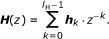(1)

The fading coefficient at delay step k is given by the complex K × NC matrix h k which describes the multi-user interferences; lH is the length of the channel impulse response. Throughout this article, we assume that the transmitter has full channel state information (CSI).

In order to equalize the temporal interferences OFDM using D subcarriers is applied. The remaining multi-user interferences at each subcarrier, described by the flat fading channel matrix(2)

have to be equalized by transmitter-sided precoding. In the following, we compare the precoding schemes (sorted) Tomlinson-Harashima Precoding ((s)THP)  with its lattice-reduction-aided variant (LRA-THP) [15, 16].

The complex-valued modulation symbols for each user k and each subcarrier d are drawn from an M-ary QAM constellation (modulation alphabet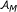) and collected in the K × D matrix A = [A k,d ], which is denoted as the frequency-domain MIMO OFDM frame. The precoding of the multi-user interferences has to be applied over the columns (vectors A d = [A(k = 1,... ,K ) ,d ], d = 1, . . . , D) of A.

The resulting precoded frequency-domain MIMO OFDM frame is denoted by the matrix X. The time-domain MIMO OFDM frame (matrix x) is obtained via an inverse discrete Fourier transform (IDFT)  along each row (vectorsof the matrix X.

Due to the D-wise superposition of the precoded frequency-domain symbols within the Fourier transform, the time-domain symbols x = [x k , d ] exhibit a large dynamic range, i.e., the peak-to-average power ratio (PAR) of these symbols is very high. As usual in literature we consider the worst-case PAR to be the relevant criterion, i.e., the maximum PAR over all antennas within one OFDM frame, which is defined as(3)

For performance comparison of the PAR reduction schemes discussed in this article, we assess the complementary cumulative distribution function (ccdf) of the PAR, i.e., the probability that the PAR of a given OFDM frame exceeds a certain threshold PARth: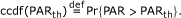(4)

Under the assumption that all samples of x are Gaussian distributed (which is a very good approximation due to the central limit theorem) and under the assumption that the samples of x are statistically independent, the ccdf of the original signal can be calculated to(5)

## Precoding Strategies

Subsequently, we consider Tomlinson-Harashima precoding  to preequalize the multi-user interferences caused by the channel in each subcarrier. The basic block diagram of this scheme, which has to be applied to each subcarrier, is depicted in Figure 1.

First, the signal vector A d (d th column of A) is passed through one of the matrices Popt,dor Zopt,d. The matrix Popt,ddescribes a permutation matrix, which is used with sorted THP. The matrix Zopt,ddescribes the unimodulara basis change matrix, which is present in LRA-THP. A detailed description how these matrices are chosen is given subsequently.

Next, the signal is precoded within the feedback-loop, i.e., it is successively processed by the feedback matrix B d , a lower triangular matrix with unit main diagonal, taking the interferences of already encoded users into account. Then the signal is modulo reduced onto the support of. After that, the signal vector is passed through the feedforward matrix F d . In order to ensure constant sum power at each subcarrier, the signal is multiplied with the scalar β d . This scalar factor is given by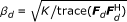.

At the receiver, the signals are scaled suitably, quantized with respect to the lattice of the constellation alphabet, and modulo reduced onto the support of. Due to the assumed scaling each user exhibits the same signal-to-noise ratio and therefore the same error performance.

### Sorted Tomlinson-Harashima precoding

When considering sorted THP the precoding order of the users is optimized in each subcarrier via the permutation matrix Popt,d. A reasonable optimization criterion is to achieve least average error rate. This is achieved in an almost optimum way if the user exhibiting the lowest signal-to-noise ratio is encoded first (reverse V-BLAST orderingb ). Considering the uplink-downlink duality, e.g., , the calculation of the optimum permutation order and the decomposition into feedforward and feedback matrix can hence be performed applying the V-BLAST algorithm  or one of its low complex implementations [21, 22]. The resulting decomposition of the channel matrix H d reads(6)

### Lattice-reduction-aided Tomlinson-Harashima precoding

In order to significantly enhance the error performance of the transmission scheme, it is possible to extend sorted THP to lattice-reduction-aided THP (LRA-THP) [15, 16]. The huge advantage of this scheme is that it achieves full diversity (here: diversity order NC), i.e., the error performance is close to that of the optimum approach of vector precoding [23, 24].

Applying a suited lattice reduction algorithm, e.g., the LLL algorithm , it is possible to decompose the channel matrix into a reduced channeland a unimodular matrixaccording to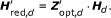(7)

The reduced channel matrixis then passed to the V-BLAST algorithm, which, including its sorting, leads a decomposition according toc(8)

Considering the precoding structure according to Figure 1, after processing the data vector with Zopt,dthe symbols are still drawn from the underlying integer grid. The following precoding equalizes the interferences caused by the reduced channel Hred,d. To this end, the aim of the LLL algorithm is to find a suited representation of the lattice spanned by the rows of H d . This representation, given by Hred,d, should fulfill two properties. On the one hand, the basis vectors should be as short as possible, on the other hand, the vectors should be close to orthogonal. Since Zopt,dchanges the lattice basis from H d to Hred,dit is also denoted as basis change matrix subsequently. A detailed analysis of this type of precoding scheme can be found in [11, 16].

## Par reduction in point-to-multipoint scenarios

### Review of selected mapping in multi-antenna environments

In the literature, selected mapping (SLM)  is one of the most popular techniques for PAR reduction in OFDM systems. The idea behind this scheme is, given the original OFDM frame, to generate several, say USLM, different signal representations via USLM different bijective mappings. Out of these signal candidates, the best one, i.e., the one exhibiting the lowest PAR, is chosen for transmission. At the receiver, after equalization the original data can be reconstructed by inverting the applied mapping. Hence, side information, in terms of an index of the applied mapping, has to be transmitted. The required redundancy has to be encoded with at least log2(USLM) bits (·: round towards plus infinity). However, this index is extraordinarily sensitive to transmission errors as the application of the wrong inverse mapping leads to the loss of the whole OFDM frame. Possible schemes to transmit the side information have been discussed in .

Originally, SLM has been proposed for single-antenna schemes. A first extension for multi-antenna point-to-point scenarios has been presented in  and named ordinary SLM (oSLM). However, this approach is nothing else than a straightforward application of single-antenna SLM to each transmit antenna. A more sophisticated extension has been presented in [8, 9] and named directed SLM (dSLM). Following the analytical analysis of these schemes in , this approach offers very promising results in terms of PAR reduction performance compared to the ordinary SLM.

### Simplified selected mapping

However, both extensions, ordinary and directed SLM, are not applicable in the multi-user point-to-multipoint scenario considered in this article. Due to the required precoding at the transmitter side, it is not possible to influence the data streams at each antenna individually. Hence, to generate different signal candidates, we have to consider the data signals of all users jointly. The corresponding extension of SLM has been originally proposed in  and named simplified SLM (sSLM).

With sSLM the original frequency-domain MIMO OFDM frame A has to be mapped jointly onto USLM different signal representations, whereby each row of A has to be mapped in the same way. Afterwards, each of the resulting signal candidates has to be precoded and transformed into time domain. Out of these, the best one, i.e., the one exhibiting the lowest PAR, is chosen for transmission.

Assuming the individual signal candidates to be statistically independent, the ccdf of sSLM can be given with respect to the ccdf of the original signal (5) and reads [7, 9](9)(10)

Subsequently, we consider this ccdf as reference for the PAR reduction performance.

### Selected sorting

Another approach to generate different signal representations, named selected sorting (SLS), has been proposed in [12, 13]. This approach combines mapping and precoding by applying different sortings in each subcarrier. In particular, different instances of THP are generated by considering different permutations of the users in each subcarrier. A practical advantage of this approach is that no side information needs to be communicated to the receiver.

The idea of SLS is as follows. A set of V different permutation matrices, v = 1,...,V, out of the set of K! possible ones are arbitrarily chosend. Starting with the optimum sorting order, we consider the alternative permutation according to(11)

Next, the information carrying signal A is precoded via all V different precoder instances and the resulting precoded signals are denoted as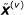, v = 1,...,V. In oder to generate USLS different signal candidates X(u), u = 1, . . . , USLS, the respective columns (corresponding to the carriers) ofare combined in USLS different ways. Hence, every column of each of the USLS signal candidates X(u)is drawn from one of the V possible precoded signals. This is possible as the actual choice of the sorting order of THP at the d th subcarrier influences the precoded signal only at this position.

Noteworthy, with this approach we are able to generate (much) more signal candidates than precoded candidates are present (USLS V may hold). The principal strategy how the USLS signal candidates are generated is depicted in Figure 2.

Moreover, SLS requires much less computational complexity compared to sSLM as the precoding has to be performed only V times to generate the USLS signal candidates. However, to further reduce the computational complexity the SLS technique could only be applied on a subset of DiD (randomly chosen) influenced subcarriers. All other subcarriers remain unaffected and the optimum sorting order is applied. Following the results of , operating only on a subset of subcarriers leads to a poor PAR reduction performance compared to the case when operating on all subcarriers. For this reason, we subsequently consider only the case for Di = D.

Compared to sSLM, assuming perfect transmission of the side information, this scheme will exhibit a small loss in error performance as suboptimal sorting orders are used to generate the signal candidates. However, even if very efficient schemes exist for transmitting the side information (e.g., ), perfect transmission is never possible. Moreover, the transmission of the side information and the inversion of the actual applied mapping requires additional signal processing at the receiver, which is not required in SLS.

### Selected basis

The idea of generating signal candidates with selected sorting may straightforwardly be extended to the case of LRA-THP as well, where the pure permutation is replaced by an unimodular matrix Zopt,d. Consequently, in this case we introduce an additional unimodular matrix. The effective unimodular basis change matrix in the d th subcarrier now reads(12)

In principal,can be chosen to be any unimodular matrix. In the following, we construct arbitrary unimodular matrices by multiplying an upper and a lower triangular matrix(13)

To guarantee that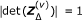, for the diagonal elements of both matriceshas to hold. Moreover, in order to ensure thatcontains only Gaussian integers, all non-zero elements of the upper and lower triangular matrix have to be Gaussian integers as well. For practical reasons we additionally restrict the magnitude of the elements, i.e.,Subsequently, we choose zmax = 1.

## Numerical results

For the subsequent numerical results, we consider transmission over an (lH = 5)-tap equal gain Rayleigh fading channel. Moreover, we assume NC = K = 4 and OFDM applying D = 512 subcarriers (all of them are active). As modulation alphabet, we consider (M = 4)-ary QAM.

## Discussion

Figure 3 shows numerical results when considering SLS as PAR reduction scheme--hence sTHP as precoding procedure. The left plot shows the respective ccdf of PAR and the right plot shows the bit error rates. The ccdf curves for Gaussian signaling ((5) or (10), depicted in gray) serve as reference.

When considering the error performance of SLS, we can observe a little loss compared to the original signal, where the optimum permutation order is applied in each subcarrier. Noteworthy, using sorted THP the diversity order is only one.

Figure 4 shows the numerical results for the PAR reduction scheme SLB--hence LRA-THP as precoding procedure. The first row of this figure displays the results for using arbitrary additional unimodular matrices according to the construction method from section "Selected basis" (zmax = 1). In terms of PAR reduction performance, the ccdf of the original signal coincides with the reference (5) and the same holds when applying SLB with USLB = 8 or USLB = 16 candidates. Hence, with LRA-THP, the effect due to the power loading over the users is not an issue as it is in sTHP. However, when considering the error performance of this approach, it is obvious that a large loss compared to original LRA-THP is present, even if a significant gain compared to sTHP is achieved.

### Choosing suited alternative precoders

As can be seen from the numerical results of Figure 4, SLB offers excellent results in terms of PAR reduction performance but also a significant loss in terms of error performance. The reason for this behavior is due to the arbitrary choice of the additional unimodular matrices. Applying such additional matrices leads to a non-optimum decomposition (with respect to the definition of LLL reduced) of the channel matrices in each subcarrier, which in turn leads to the significant loss of the error rate. However, applying arbitrary additional unimodular matrices, it is possible to generate statistical independent signal candidates which leads to a PAR reduction performance equal to the reference (9).

Subsequently, we study the influence of the additional unimodular matrix. Starting point is the decomposition (8), where the channel matrix of the d th subcarrier is decomposed into the unimodular matrix Zopt,dand the reduced matrix. Now, if an additional unimodular matrixis applied, the effective reduced channel and its QR-type decomposition reads(14)

The idea of the LLL algorithm is to find a more suited representation (Hred,d) of the lattice spanned by the rows of the channel matrix H d . Thereby, the row vectors of Hred,dshould be as short as possible and close to orthogonal. Applying the additional unimodular matrix, this property remains also valid foras long asis unitary.

As a first approach, this can be achieved when allowing only pure permutation matrices for, similar to the SLS approach.

The second row of Figure 4 shows numerical results for this case. Now, there is no loss in terms of error ratios compared to the original signal. However, the ccdf curves flatten out. The reason for this effect is that the restriction to pure permutation matrices offers not enough degrees of freedom to generate statistical independent signal candidates.

In order to introduce more degrees of freedom but ensure that the additional unimodular matricesare still unitary, we allow matrices containing exactly one element from the set {±1, ±j} in each row and column and only zeros at all other positions. Such matrices are a generalization of permutation matrices and subsequently denoted as permutation/phase matrices. In total, there exist exactly 4K K! of such matrices.

The bottom row of Figure 4 shows numerical results when using such unimodular matrices to generate alternative signal candidates. It can be seen, that there is no loss in terms of error rates again. Additionally, the flattening of the ccdf curves is significantly reduced compared to the case when using pure permutation matrices. The PAR reduction performance when allowing arbitrary unimodular matrices can almost be achieved. Hence, with this kind of matrices it is possible to offer sufficient degrees of freedom to generate almost statistical independent signal candidates.

### Analysis of computational complexity

As already mentioned above, the PAR reduction/precoding schemes SLS and SLB have two major advantages compared to sSLM. On the one hand, no side information has to be transmitted and, on the other hand, the computational complexity is reduced, as the precoding procedure has to be performed only V times to generate USLS/SLB > V signal candidates. In the following, we compare the PAR reduction performancee of sSLM with the schemes SLS and SLB, respectively, incorporating the computational complexity. In this context, as complexity measure we consider the number of complex operations and treat multiplications and divisions equally. However, additions and multiplications with Gaussian integers are not incorporated into the counting.

In the following, we assume that the channel remains constant for the duration of NB OFDM symbols. Hence, for this block of OFDM symbols the calculation of the precoding matrices has to be performed only once, whereas the computation of the precoded signal, the FFT, and the selection metric have to be accomplished for each of the NB OFDM symbols.

With SLS or SLB, the computational complexity (per carrier) consists of the single calculation of the optimum decomposition (factorization) of the channel matrix according to (6) or (8). This complexity is denoted as cfac. In addition to that, V - 1 alternative precoding matrices have to be determined. For each alternative, the computational complexity cQR of one QR-decomposition  is needed.

The V alternative precoders are now valid for NB OFDM blocks. For each of these OFDM blocks, we have to precode the MIMO OFDM frame V times. Moreover, USLS/SLBK calculations of the inverse Fourier transform (complexity cFFT) and of the selection metric (complexity cmet) are necessary in order to determine the best signal candidate.

Using sSLM, the complexity consists also of the calculation of the optimum decomposition of the channel (complexity cfac) and of USLMK transformations into time-domain (complexity cFFT) and PAR evaluations (complexity cmet). Generating the different signal candidates is not incorporated into the considerations, as it is implemented via the multiplication of phase vectors (cf. ) and different candidates differ only in a change of sign or interchange of the quadrature components of the QAM symbols within each subcarrier. This operation is trivial in terms of computational complexity. Finally, the precoding of the signal has to be applied for each of the USLM signal candidates.

In summary, the computational complexities of SLS/SLB and sSLM sum up to(15)(16)

For a fair comparison of sSLM with SLS or SLB, the respective scheme should exhibit the same complexity (i.e., cSLS/SLB csSLM). Given the parameters V and USLS/SLB for SLS or SLB then sSLM assessing(17)

signal candidates will exhibit approximately the same computational complexity. Hereby, when rounding the number USLM of assessed candidates for sSLM to the next greater integer, sSLM will exhibit a slightly larger complexity.

In order to evaluate this number, we have to further specify the complexities cQR, cprec, cFFT, and cmet. The calculation of the feedforward and feedback matrices is usually implemented via a QR-type decomposition  and requires(18)

complex operations. The precoding of the transmit signal requires(19)

complex operations; the transformation into time domain (implemented as fast Fourier transform ) and the calculation of the decision metric (PAR) require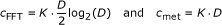(20)

complex multiplications, respectively.

For the following numerical results we choose the block lengths NB = 10 and fix the number of assessed signal candidates for SLS or SLB to either USLS/SLB = 8 or USLS/SLB = 16. The respective numbers of assessed signal candidates for sSLM according to (17) will be USLM = 7 and USLM = 11.

Figure 5 shows the ccdf of PAR of sSLM and SLS. In this case, sSL M outperforms SLS even if less signal candidates are assessed. The reason for this behavior is that SLS is not able to generate statistical independent signal candidates as it is possible with sSLM. Hence, the ccdf curves of SLS flatten out compared to sSLM, which leads to the worse performance.

Numerical results of the comparison of sSLM with SLB are depicted in Figure 6. The top plot shows the results when using arbitrary unimodular matrices (cf. section "Selected basis"). In this case, sSLM is outperformed by SLB in terms of PAR reduction. However, cf. Figure 4, when choosing arbitrary unimodular matrices in SLB the loss in error rate compared to the original signal is significant.

The middle plot of Figure 6 compares the PAR reduction performance when restricting the additional unimodular matrices in SLB to permutation matrices. Now, it is no longer possible to generate statistical independent signal candidates, which leads to some flattening of the ccdf curves. Hence, SLB is outperformed by sSLM due to the steeper ccdf curves.

The bottom plot shows results when applying permutation/phase matrices for the additional unimodular matrices. In this case, the PAR reduction performance of SLB is more or less equal to the one of sSLM. Additionally, according to the numerical results of Figure 4, the loss in terms of bit error ratios is negligible. Noteworthy, the huge benefit of S LB is that no side information has to be communicated and no error multiplication due to erroneous side information occurs as it would with sSLM.

## Conclusions

This article introduces a novel combined precoding/PAR reduction scheme for OFDM multi-user downlink scenarios. This scheme, named selected basis (SLB), is a further development of the scheme selected sorting (SLS). Both schemes are based on the idea of generating multiple redundant signal representations and selecting the one exhibiting the lowest PAR and are thus based on the philosophy of the SLM family. The multiple signal representations are generated by applying different instances of the precoder, which has to be applied within the multi-user downlink scenario. In particular, SLS generates multiple instances of the precoder by applying different permutations within the Tomlinson-Harashima precoding scheme. SLB works in combination with LRA precoding and generates different instances of the precoder by employing different additional unimodular (basis change) matrices. It turns out that the best PAR reduction performance can be achieved when using arbitrary unimodular matrices as an offset to the optimum (with respect to the definition of LLL reduced) basis change matrix. However, the error performance is quite poor in this case. The best trade-off between PAR reduction capabilities and error performance can be achieved when restricting the additional unimodular matrices to so-called permutation/phase matrices.

Finally, the PAR reduction performance of SLS and SLB is compared with the one of sSLM, the only feasible extension of SLM for the multi-user downlink scenario. For a fair comparison, the parameter of both schemes are chosen that they exhibit (almost) the same computational complexity. It turns out that sSLM offers better PAR reduction performance than SLS, because it is not possible to generate statistical independent signal candidates with SLS but with sSLM. However, the PAR reduction performance of SLB is almost the same as that of sSLM. Noteworthy, the huge benefit of SLS and SLB is that in contrast to sSLM no side information has to be communicated to the receiver. It can be summarized that using SLB in the OFDM multi-user downlink, both, very good PAR statistics and full diversity error performance can be achieved. As the receivers do not require any side information, it is a very attractive strategy for future downlink transmission systems.

## Endnotes

aA unimodular matrix Z = [zm,n] contains only Gaussian integers, i.e., all elements zm,nare from the setand for its determinant |det(Z)| = 1 has to hold.

bThe V-BLAST algorithm calculates the optimum detection order for decision-feedback equalization when transmitting over MIMO channels.

cThe LLL algorithm can directly perform the decomposition (8) of the channel matrix H d into the unimodular matrix Zopt,d, the feed forward matrix F d , and the feedback matrix B d . However, no explicit control on the resulting sorting is possible in this case.

dIn principal, it is reasonable to select V additional permutation matrices out of the set of K! ones, which have only marginal influence on the error ratio. Such a suited choice is discussed in , where only additional permutation matrices are used which do not change the encoding position of the last encoded user (with respect to the optimum sorting order). This strategy makes sense because no power loading of the users is applied in . On the contrary, in this paper, power loading over the users is applied (cf. Figure 1), which makes the selection of suited additional permutation matrices not that easy. However, according to the numerical results shown in Sec., choosing arbitrary additional permutation matrices exhibits almost the same performance as the optimum permutation, which makes this strategy a reasonable approach.

eIn this paper, the comparison of sSLM with SLS or SLB, respectively, is done in terms of the PAR reduction performance. Comparing also the error performance of the respective schemes needs to incorporate a specific strategy to transmit the side information with sSLM. Certainly, the exist a wide range of different schemes to transmit the side information for the original approach of SLM (cf. [2729, 3234]), which can be easily transferred to sSLM as well. Some of these schemes are able to transmit the side information very reliable. For the sake of brevity, we do not consider a specific scheme and omit the comparison of the error performance in this paper. Noteworthy, even if a reliable transmission of the side information with sSLM is possible, error propagation will still occur. Moreover, the transmission of the side information leads to additional complexity within transmitter and receiver. This additional complexity is not required with SLS or SLB, which is a further advantage of these schemes.

## Abbreviations

ACE:

active constellation extension

CSI:

channel state information

LRA-THP:

lattice-reduction-aided variant

MIMO:

multiple-input/multiple-output

OFDM:

orthogonal frequency-division multiplexing

PTS:

partial transmit sequences

PAR:

peak-to-average power ratio

SLB:

scheme selected basis

SLS:

scheme selected sorting

sSLM:

simplified selected mapping

sTHP:

sorted Tomlinson-Harashima precoding

TR:

tone reservation.

## References

1. 1.

Bingham JAC: Multicarrier modulation for data transmission: an idea whose time has come. IEEE Commun Mag 1990, 5-14.

2. 2.

Bäuml R, Fischer RFH, Huber JB: Reducing the peak-to-average power ratio of multicarrier modulation by selected mapping. IEE Electron Lett 1996,32(22):2056-2057. 10.1049/el:19961384

3. 3.

Müller S, Huber JB: OFDM with reduced peak-to-average power ratio by optimum combination of partial transmit sequences. IEE Electron Lett 1997,33(5):368-369. 10.1049/el:19970266

4. 4.

Krongold BS, Jones DL: PAR Reduction in OFDM via Active Constellation Extension. IEEE Trans Broadcast 2003,49(3):258-268. 10.1109/TBC.2003.817088

5. 5.

Tellado J: Peak to Average Power Reduction for Multicarrier Modulation. PhD thesis. Stanford University; 2000.

6. 6.

Telatar E: Capacity of multi-antenna gaussian channels. Eur Trans Telecommun 1999,10(6):585-596. 10.1002/ett.4460100604

7. 7.

Baek M-S, Kim M-J, You Y-H, Song H-K: Semi-Blind Channel Estimation and PAR Reduction for MIMO-OFDM System with Multiple Antennas. IEEE Trans Broadcasting 2004,50(4):414-424. 10.1109/TBC.2004.837885

8. 8.

Fischer RFH, Hoch M: Directed selected mapping for peak-to-average power ratio reduction in MIMO OFDM. IEE Electron Lett 2006,46(22):1289-1290.

9. 9.

Fischer RFH, Hoch M: Peak-to-average power ratio reduction in MIMO OFDM. Proceedings of IEEE International Conference on Communications (ICC), Glasgow, Scotland 2007.

10. 10.

Fischer RFH: Precoding and Signal Shaping for Digital Transmission. Wiley, New York; 2002.

11. 11.

Windpassinger C: Detection and Precoding for Multiple Input Multiple Output Channels. PhD thesis. Universität Erlangen-Nürnberg; 2004.

12. 12.

Siegl C: RFH Fischer, Peak-to-average power ratio reduction in multi-user OFDM. Proceedings IEEE International Symposium on Information Theory (ISIT). Nice, France 2007.

13. 13.

Siegl C: RFH Fischer, Selected Sorting for PAR Reduction in OFDM Multi-User Broadcast Scenarios. Proceedings of International ITG/IEEE Workshop on Smart Antennas, Berlin, Germany 2009.

14. 14.

van Trees RG: Detection, Estimation, and Modulation Theory-Part III: Radar-Sonar Signal Processing and Gaussian. In Signals in Noise. Wiley, New York; 1971.

15. 15.

Windpassinger C: RFH Fischer, JB Huber, Lattice-reduction-aided broadcast precoding. IEEE Trans Commun 2004,52(12):2057-2060. 10.1109/TCOMM.2004.838732

16. 16.

Stierstorfer C, Fischer RFH: Lattice-reduction-aided tomlinson-harashima precoding for point-to-multipoint transmission. Int J Electron Commun (AEU) 2006, 60: 328-330. 10.1016/j.aeue.2005.08.002

17. 17.

Oppenheim AV, Schafer RW: Discrete-Time Signal Processing. Prentice-Hall, Upper Saddle River; 1999.

18. 18.

Fischer RFH, Siegl C: Peak-to-Average Power Ratio Reduction in Single- and Multi-Antenna OFDM via Directed Selected Mapping. IEEE Trans Commun 2009,11(11):3205-3208.

19. 19.

Viswanath P, Tse DNC: Sum capacity of the vector gaussian broadcast channel and uplink-downlink duality. IEEE Trans Inf Theory 2003,49(8):1912-1922. 10.1109/TIT.2003.814483

20. 20.

Wolniansky PW, Foschini GJ, Golden GD, Valenzuela RA: V-BLAST: An architecture for realizing very high data rates over the rich-scattering wireless channel. URSI International Symposium on Signals, Systems, and Electronics, Pisa, Italy 1998, 295-300.

21. 21.

Wübben D, Rinas J, Böhnke R, Kühn V, Kammeyer KD: Efficient algorithm for detecting layered space-time codes. Proceedings of 4th International ITG Conference on Source and Channel Coding (SCC), Berlin, Germany 2002.

22. 22.

Benesty J, Huang Y, Chen J: A Fast Recursive Algorithm for Optimum Sequential Signal Detection in a BLAST System. IEEE Trans Signal process 2003,51(7):1722-1730. 10.1109/TSP.2003.812897

23. 23.

Schmidt D, Joham M, Utschick W: Minimum mean square error vector precoding. Proc PIMRC '05 2005.

24. 24.

Taherzadeh M, Mobasher A, Khandani AK: Communication over MIMO broadcast channels using lattice-basis reduction. IEEE Trans Inf Theory 2007,53(12):4567-4582.

25. 25.

Lenstra AK, Lenstra HW, Lovász L: Factoring polynomials with rational coefficients. Math Ann 1982, 261: 515-534. 10.1007/BF01457454

26. 26.

Breiling M, Müller-Weinfurtner S, Huber JB: SLM peak-power reduction without explicit side information. IEEE Commun Lett 2001,5(6):239-241. 10.1109/4234.929598

27. 27.

Khoo BK, Le Goff SY, Tsimenidis CC, Sharif BS: OFDM PAPR Reduction Using Selected Mapping Without Side Information. Proceedings of IEEE International Conference on Communications (ICC), Glasgow, Scotland 2007.

28. 28.

Siegl C, Fischer RFH: Selected mapping with implicit transmission of side information using discrete phase rotations. Proceedings of 8th International ITG Conference on Source and Channel Coding (SCC), Siegen, Germany 2010.

29. 29.

Siegl C, Fischer RFH: Selected Mapping with Explicit Transmission of Side Information. Proceedings of IEEE Wireless Communication and Networking Conference (WCNC), Sydney, Australia 2010.

30. 30.

Golub GH, Van Loan CF: Matrix Computations. The Johns Hopkins University Press, Baltimore; 1996.

31. 31.

Wübben D, Böhnke R, Kühn V, Kammeyer K-D: Near-maximum-likelihood detection of MIMO systems using MMSE-based lattice reduction. Proceedings of IEEE International Conference on Communications (ICC) 2004.

32. 32.

Jaylath ADS, Tellambura C: SLM and PTS peak-power reduction of OFDM signals without side information. IEEE Trans Wireless Commun 2005,4(5):2006-2013.

33. 33.

Baxley RJ, Zhou GT: MAP metric for blind phase sequence detection in selected Mapping. IEEE Trans Broadcasting 2005,51(4):565-567. 10.1109/TBC.2005.854170

34. 34.

Alsusa E, Yang L: Redundancy-free and BER-maintained selective mapping with partial phase-randomising sequences for peak-to-average power ratio reduction in OFDM systems. IET Commun 2008,2(1):66-74. 10.1049/iet-com:20070055

## Acknowledgements

This work was supported in parts by Deutsche Forschungsgemeinschaft (DFG) within the frame-work TakeOFDM under grant FI 982/1-2.

## Author information

Authors

### Corresponding author

Correspondence to Christian Siegl.

### Competing interests

The authors declare that they have no competing interests.

## Authors’ original submitted files for images

Below are the links to the authors’ original submitted files for images.

## Rights and permissions

Reprints and Permissions

Siegl, C., Fischer, R.F. Selected basis for PAR reduction in multi-user downlink scenarios using lattice-reduction-aided precoding. EURASIP J. Adv. Signal Process. 2011, 17 (2011). https://doi.org/10.1186/1687-6180-2011-17